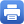Columbia Home
Analysis and Probability

Students select Analysis and Probability I in the fall semester, and then pick a specialization in the spring; either Analysis II or Probability II.

### Analysis and Probability I

I Measure Theory

• Construction of the integral, limits and integration
• Lp spaces of functions
• Construction of measures, Lebesgue-Stieltjes product measures
• Examples: ergodicity, Liouville measure, Hausdorff measure

II Elements of Probability

• The coin-tossing or random walk model
• Independent events and independent random variables
• The Khintchin weak law and the Kolmogorov strong law of large numbers
• Notions of convergence of random variables
• The Central Limit Theorem

III Elements of Fourier Analysis

• Fourier transforms of measures, Fourier-Lévy Inversion Formula
• Convergence of distributions and characteristic functions
• Proof of the Central Limit and Lindeberg Theorems
• Fourier transforms on Euclidean spaces
• Fourier series, the Poisson summation formula
• Spectral decompositions of the Laplacian
• The heat equation and heat kernel

IV Brownian Motion

• Brownian motion as a Gaussian process
• Brownian motion as scaling limit of random walks
• Brownian motion as random Fourier series
• Brownian motion and the heat equation
• Elementary properties of Brownian paths

### Analysis II: Partial Differential Equations and Functional Analysis

I First Order Partial Differential Equations

• Cauchy’s Theorem for first order real partial differential equations
• Completely integrable first order equations

II Implicit Function Theorems

• Basic examples of linear and non-linear partial differential equations
• The functional analytic framework, Banach and Hilbert spaces
• Bounded linear operators, spectrum, invertibility
• Implicit function theorems in Banach spaces
• Sketch of subsequent applications to the basic examples

III Second Order Partial Differential Equations

• Qualitative description: elliptic,parabolic, hyperbolic equation
• The Cauchy problem
• Maximum principles
• Sobolev and Schauder spaces
• A priori estimates and Green’s functions
• Riesz-Schauder theory of compact operators
• Detailed treatment of basic examples
• The Laplace and heat equations on compact manifolds
• Applications to de Rham and Hodge theory

IV Selected Topics, chosen from

• Riemann-Roch and index theorems
• Determinants of Laplacians, modular forms
• Integral representations, Hilbert transforms, singular integral operators
• Subelliptic equations
• Nash-Moser implicit function theorems
• Non-linear equations from geometry or physics

### Probability II

Prerequisite: Analysis and Probability I.  Can be taken concurrently with Analysis II.

I Rare Events

• Cramér’s Theorem
• Introduction to the Theory of Large Deviations
• The Shannon-Breiman-McMillan Theorem

II Conditional Distributions and Expectations

• Absolute continuity and singularity of measures
• Conditional expectations as least-square projections
• Notion of conditional independence
• Introduction to Markov Chains. Harmonic functions

III Martingales

• Definitions, basic properties, examples, transforms
• Optional sampling and upcrossings theorems, convergence
• Burkholder-Gundy and Azuma inequalities
• Doob decomposition, square-integrable martingales
• Strong laws of large numbers and central limit theorems

IV Applications

• Optimal stopping
• Branching processes and their limiting behavior. Urn schemes
• Stochastic approximation. Probabilistic analysis of algorithms

V Stochastic Integrals and Stochastic Differential Equations

• Detailed study of Brownian motion
• Martingales in continuous time
• Doob-Meyer decomposition, stopping times
• Integration with respect to continuous martingales, Itô’s rule
• Girsanov’s theorem and its applications
• Introduction to stochastic differential equations. Diffusion processes

VI Elements of Potential Theory

• The Dirichlet problem. Poisson integral formula
• Solution in terms of Brownian motion
• Detailed study of the heat equation; Cauchy and boundary-value problems
• Feynman-Kac theorems, applicationsPrint this page